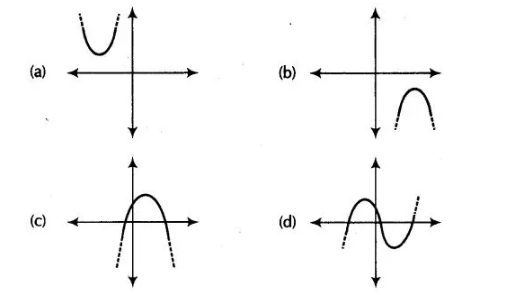# Which of the following is not the`
Question:

Which of the following is not the graph of a quadratic polynomial?Solution:

(d) For any quadratic polynomial ax2 + bx + c, a≠0, the graph of the Corresponding equation y = ax2 + bx + c has one of the two shapes either

open upwards like u or open downwards like ∩ depending on whether a > 0 or a < 0. These curves are called parabolas. So, option (d) cannot be

possible.

Also, the curve of a quadratic polynomial crosses the X-axis on at most two points but in option (d) the curve crosses the X-axis on the three

points, so it does not represent the quadratic polynomial.